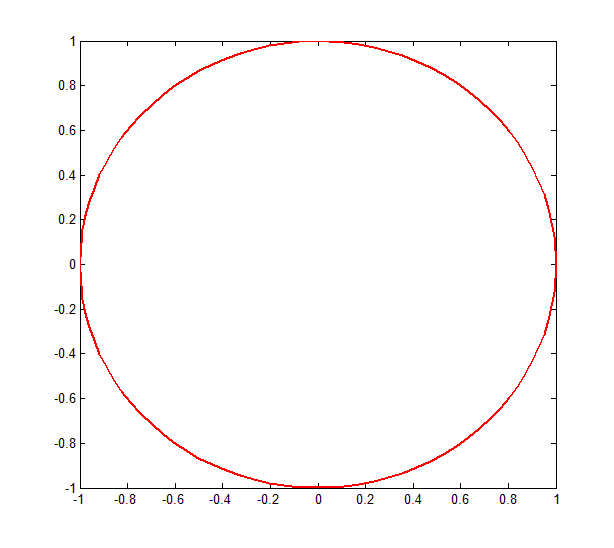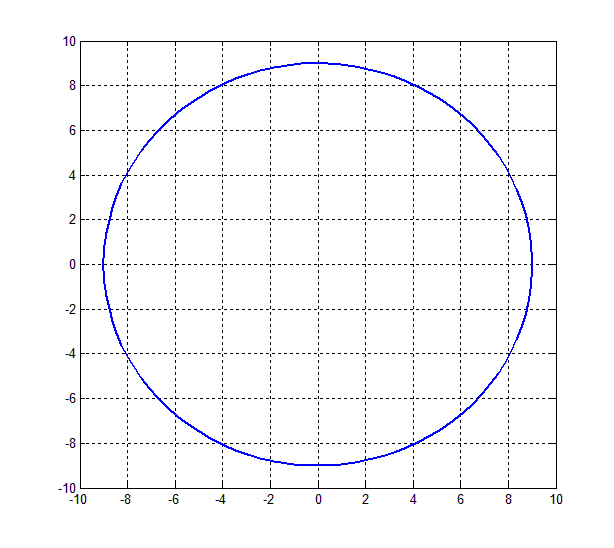How to plot a circle in Matlab | 12CAD.com

# How to plot a circle in Matlab

Here is  how to plot a circle in Matlab

### Plot the unity circle in Matlab

Code

```Angles=(0,2*pi,100);
plot(cos(angles), sin(angles))```### Plot a circle in Matlab with a given radius

```Angles=(0,2*pi,100);
plot(9*cos(angles), 9*sin(angles))```